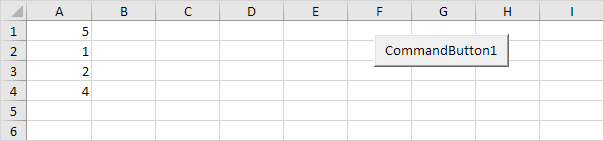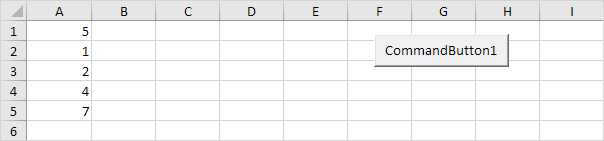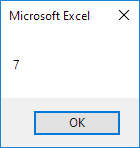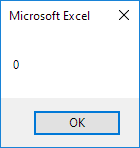# Dynamic Array

If the size of your array increases and you don't want to fix the size of the array, you can use the ReDim keyword. Excel VBA then changes the size of the array automatically.

Add some numbers to column A.Place a command button on your worksheet and add the following code lines:

1. First, we declare the array named numbers. Also declare two variables of type Integer. One named size and one named i.

Dim numbers() As Integer, size As Integer, i As Integer

Note: the array has no size yet. numbers, size and i are randomly chosen here, you can use any names. Remember to refer to these names in the rest of your code.

2. Next, we determine the size of the array and store it into the variable size. You can use the worksheet function CountA for this. Add the following code line:

size = WorksheetFunction.CountA(Worksheets(1).Columns(1))

3. We now know the size of the array and we can redimension it. Add the following code line:

ReDim numbers(size)

4. Next, we initialize each element of the array. We use a loop.

For i = 1 To size
numbers(i) = Cells(i, 1).Value
Next i

5. We display the last element of the array using a MsgBox.

MsgBox numbers(size)

6. Exit the Visual Basic Editor and click the command button on the sheet.

Result:7. Now to clearly see why this is called a dynamic array, add a number to column A.8. Click the command button again.Conclusion: Excel VBA has automatically changed the size of this dynamic array.

9. When you use the ReDim keyword, you erase any existing data currently stored in the array. For example, add the following code lines to the previously created code:

ReDim numbers(3)
MsgBox numbers(1)

Result:The array is empty.

10. When you want to preserve the data in the existing array when you redimension it, use the Preserve keyword.

ReDim Preserve numbers(3)
MsgBox numbers(1)

Result:Go to Next Chapter: Function and Sub